# ISEE Upper Level Math : How to find the area of a rhombus

## Example Questions

### Example Question #1 : How To Find The Area Of A Rhombus

Two diagonals of a rhombus have lengths of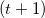and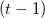. Give the area in terms of.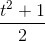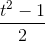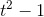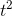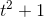Explanation:

The formula for the area of a rhombus is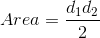,

where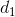is the length of one diagonal and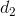is the length of the other diagonal.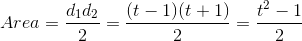### Example Question #281 : Plane Geometry

A rhombus has side length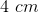, and one of the interior angles is. Give the area of the rhombus.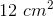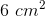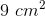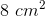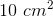Explanation:

The area of a rhombus can be determined by the following formula: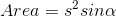,

whereis the length of any side and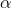is any interior angle.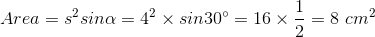### Example Question #1 : How To Find The Area Of A Rhombus

Find the area of a rhombus with one diagonal having a length of 12in and the other having a length two times the first diagonal.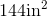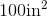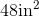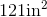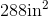Explanation:

To find the area of a rhombus, we will use the following formula: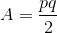where p and q are the lengths of the diagonals of the rhombus.

Now, we know one diagonal has a length of 12in.  We also know the other diagonal is two times the first diagonal.  Therefore, the second diagonal is 24in.

Knowing this, we can substitute into the formula.  We get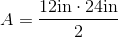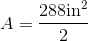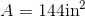### All ISEE Upper Level Math Resources